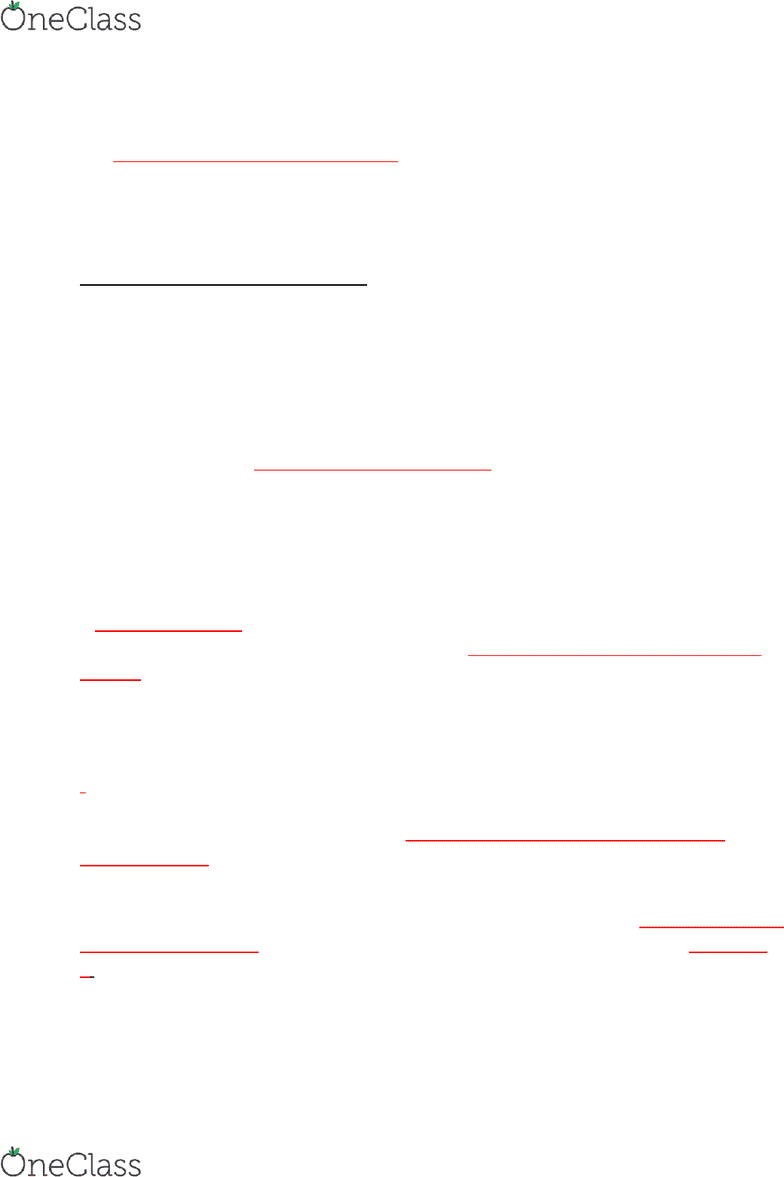Textbook Notes (280,000)
CA (170,000)
York (10,000)
MGMT (200)
Chapter 16

# MGMT 1050 Chapter 16: CH 16 Notes part1

Department
Management
Course Code
MGMT 1050
Professor
Olga Kraminer
Chapter
16

This preview shows page 1. to view the full 5 pages of the document.Chapter 16
The purpose of simple regression analysis is to predict the value of one variable based on
the value of one other variable using a mathematical equation
The variable whose value your trying to predict is the dependant variable (y), and the
variable you are using to predict is the independent variable (x)
Deterministic vs. Probabilistic Model
Deterministic model predicts a specific value for each value of the independent variable
(similar to the point estimate)
Probabilistic model same as the deterministic model but also incorporates the
randomness of real-life.
For instance, if we are trying to determine the number of pieces of candy in a bag based on
the weight of the bad, a deterministic model could be
Y = 1/3x
Where y is the number of candy in the bag and  is the ag’s eight i gras. A
deterministic model would predict that there are 30 pieces of candy in a bag that weights
90g
A probabilistic model would also account for random variation in the weight of the pieces of
candy. To make a probabilistic model, we take the deterministic model and add the error
variable
Y = 1/3x + E
Note: in this example E reps the number of candy and not the gram of the candy
E is equal to the difference between the actual value of y (the true number of pieces in the
bag of candy) and the value that the deterministic model predicts (the number of pieces of
candy in that bag that is predicted, so 30). Even if x is constant the value of the error
variable will vary. Not all bags that weigh 90g have 30 pieces of candy in them the number
of pieces of candy fluctuates, so the error variable must as well
When we make a model, we would like to be accurate as possible, so we make it so that the
sum of all the residuals (the difference between the true and predicted values) is equal to
0. Basically the difference between actual y and the sample y or y-hat. As long as the sum of
residuals is 0 we are good (the line does not have to pass through every point) last
paragraph in pg. 52
The first-order linear model (or the simple linear regression model) has the form
find more resources at oneclass.com
find more resources at oneclass.com

Unlock to view full version

Only page 1 are available for preview. Some parts have been intentionally blurred.Y = B0 +B1x + E
In this model, y is the dependant variable, x is the independent variable, B0 is the y-
intercept, and B1 is the slope of the line, while E is the error variable. Linear means that the
equation generates a straight line between the variables. BO and B1 are population
parameters that describe the relatioship etee  ad , ut the’re alost alas
unknown. Which is why the equation we normally use in stats is
̂= bO +b1x
Note that the equation above (simple linear regression model) still has an error term even
though BO and B1 are population parameters, this is because of (a) other variables that
impact the value of y and (b) random effects. We do not expect the same value of y even if
we use the same value of x because of the error variable
In a positive linear relationship, y increases as x does (the line has a positive slope). In a
negative linear relationship, y decreases as x increases (the line has a negative slope).
Horizontal lines (with a slope of 0) imply no relationship since the y value is constant
regardless of the value of x.
Estimating the Coefficients
The sample regression line below provides an estimate of the population regression line
(or the simple linear regression model above). To estimate the values of the parameters, we
do what we always do, which is to take a random sample from the population and calculate
the sample statistics. By making a satterplot of our saple data ad draig the est
straight line that comes closest to all the data points, we can guess what the true
population parameter are.
The line that minimizes the difference between itself and the observed values is called the
least squares line and has the equation
̂= bO +b1x
In this model y-hat is the predicted value of y, x is the independent variable, bo is the y-
intercept (statistic) and b1 is the slope of the line (statistic).
Note that you can use population parameters BO and B1 as long as you add a carat (^).
When you add a carat it becomes a statistic.
The least squares method chooses coefficients that create a straight line that minimizes the
sum of squared differences between the predicted points (y-hat) and the actual ones (y) in
the samples.
find more resources at oneclass.com
find more resources at oneclass.com